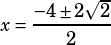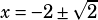Test 3 Section 3 Number 14

OK, so you’re going to be doing the quadratic formula for this one. (The question signals this to you with the answer choices—you shouldn’t even be thinking about trying to factor because all the answer choices have ± and radicals in them.)

The quadratic formula: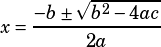So plug the values from the given quadratic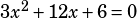in: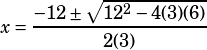WAIT A MINUTE. This is the no calculator section, right? Well, then let’s make our lives slightly easier and factor a 3 out of the quadratic first.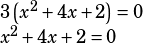Yeah, that’s better. NOW put those values into the quadratic formula: